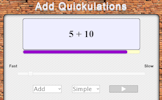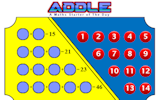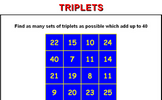# Solving problems with addition and subtraction

### Term 2 starting in week 1 :: Estimated time: 2 weeks

• Properties of addition and subtraction
• Mental strategies for addition and subtraction
• Use formal methods for addition of integers
• Use formal methods for addition of decimals
• Use formal methods for subtraction of integers
• Use formal methods for subtraction of decimals
• Choose the most appropriate method: mental strategies, formal written or calculator
• Solve problems in the context of perimeter
• Solve financial maths problems
• Solve problems involving tables and timetables
• Solve problems with frequency trees
• Solve problems with bar charts and line charts

For higher-attaining pupils:

• Add and subtract numbers given in standard form

This page should remember your ticks from one visit to the next for a period of time. It does this by using Local Storage so the information is saved only on the computer you are working on right now.

## Lesson Starters

Here are some suggestions for whole-class, projectable resources which can be used at the beginnings of each lesson in this block.

### 1st LessonCalculations appear on the screen every few seconds. This mental arithmetic starter provides pace to the start of the Maths lesson.

### 2nd LessonArrange the numbers 1 to 14 in the circles so that the sums are correct.

### 3rd Lesson#### Light Shopping

A lamp and a bulb together cost 32 pounds. The lamp costs 30 pounds more than the bulb. How much does the bulb cost?

### 4th Lesson#### Broken Calculator (4 and 5)

Which numbers can be made with the buttons which have not yet dropped off this calculator?

### 5th Lesson#### Spinsum

Arrange the numbers on the grid of squares so that the totals along each line of three squares are equal.

### 6th Lesson#### Triplets

Find as many sets of three of the available numbers as possible which add up to the given total.

Some of the Starters above are to reinforce concepts learnt, others are to introduce new ideas while others are on unrelated topics designed for retrieval practice or and opportunity to develop problem-solving skills.

White Rose ResourcesEnd of block assessments provide a quick progress check at the end of each block of learning to make sure students have understood the content covered. This Scheme of Learning was produced by White Rose Maths and is used here with permission granted on 30th June 2021.For All: